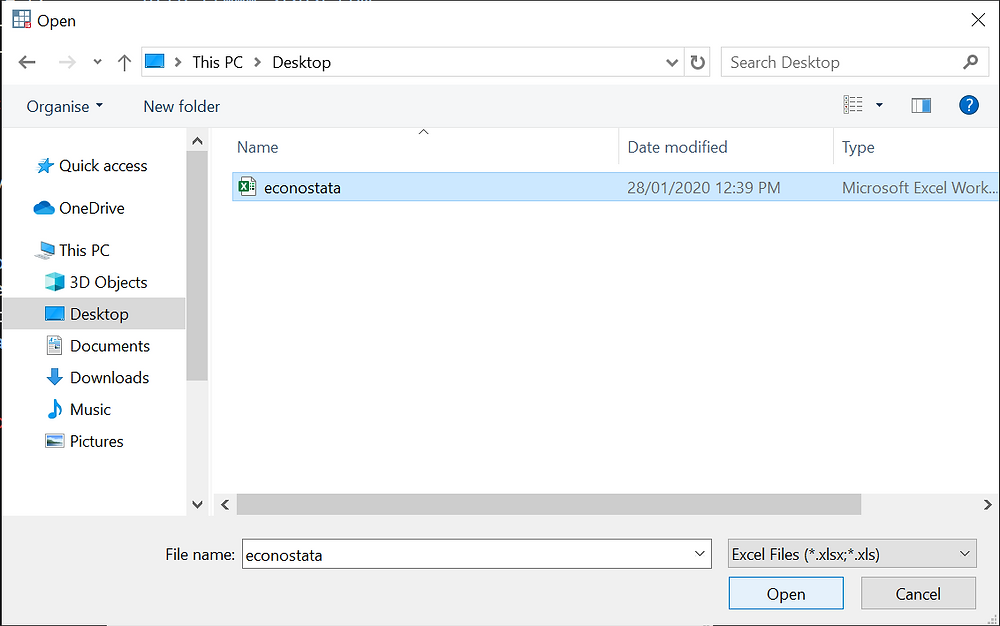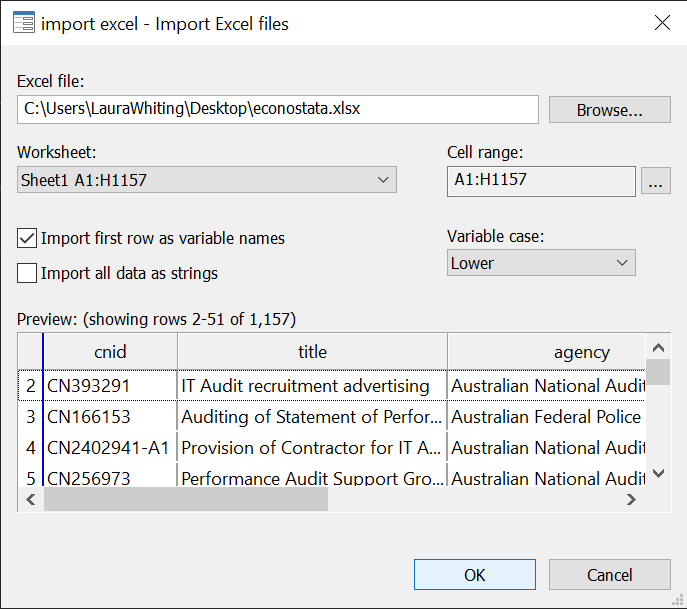Search

Getting Started in Stata - Importing Microsoft Excel Files

Data is shared in many different formats, with one of the most common file formats being Microsoft Excel (.xls or .xlsx) spreadsheets. If you have received your raw data in this format you will need to import it into Stata in order to analyse it. Here we will go through how to import an MS Excel file using both Stata menus and commands.

File > Import > Excel spreadsheet (*.xls;*.xlsx)Click Browse...Navigate to the location of the file you want to import and select it. We are going to import a dataset called econostata.xlsx.

Click OpenOnce you select your dataset you will be shown a snapshot of that dataset and how it is going to import.

By default Stata will assume the excel column names (A, B, C, etc.) are the variable names. If the first row of your spreadsheet contains the true variables names, select the tick box "Import first row as variable names" as I have done in the image above.

By default Stata will preserve the text case of the variable names. It is generally recommended that you change this from "Preserve" to "Lower" via the drop-down menu below "Variable case:" on the right side of the window, as I have done in the image above.

Note: Stata variable names are case-sensitive. If you preserve the case of your variable names you will need to remember where upper and lower case letters are in each variable name in order to use those variables in commands in Stata.

If you have multiple worksheets in your spreadsheet, they will need to be imported one at a time as separate datasets. Once imported and saved these individual sheet datasets can be merged or appended as needed. Stata will automatically assume you want to import the first sheet.

If there is another sheet you want to import, you will need to select it from the drop-down menu under "Worksheet:" on the left side of the window. In the above image the selected sheet is shown in the selection box as "Sheet1 A1:H1157".

Stata will try to detect the cell range for you, and it will select all columns and rows with data. If your spreadsheet is set up with empty columns/rows between filled columns/rows you may need to alter the cell range as Stata will stop its selection when it reaches the first empty column and row. Additionally, if you only want to import part of the spreadsheet you may want to alter the cell range to include only the information you want.

To specify your cell range, click the "..." button or simply type in your new cell range in the box below "Cell range:" on the right side of the window.

There is also an option to "Import all data as strings", which is a tick box below the tick box for "Import first row as variable names". There are some instances where you want to format or convert numeric variables in a special way in Stata. It is sometimes easier to do this by importing a numeric variable as a string and then formatting it appropriately once it is in Stata. If this is something you need to do, make sure to select this tick box. We do not want to do this for this example.

Once you have made the selections appropriate for your dataset, click OK

Your dataset should now be loaded, and you should be able to examine it using the browse command.

Import Excel using Stata Commands:

To import an excel spreadsheet through the Command pane you use the command import excel. There are several options that can be added to this command as needed. To import the same spreadsheet in the same way as was done above with the menus, you use the following command:

In the command above, you start with the command import excel followed by the full file path of the excel file you are importing. You then add a comma, and anything after the comma are options you need to specify if you want to alter the default import settings.

The sheet() option used in this command gives the name of the sheet you are importing. If there is only one sheet in the file you do not need to specify this option.

The firstrow option used in this command tells Stata that the first row of your spreadsheet contains the variable names.

The case() option used in this command tells Stata which case the variable names should be imported as. You can specify either lower, upper, or preserve inside the brackets. It is generally recommended to specify the case as lower when importing, as was done in our command above.

There are additional options you can add which we did not use here. Any option just needs to be added after the comma, as was done in the command above. Make sure to separate multiple options with a space between each option. The additional options not used here are outlined below as follows:

The cellrange() option is used when you want to specify a range that is different to what Stata has selected by default. You can specify a cell range in one of two ways. First, you can just give the starting (top left) cell for your dataset. For example - cellrange(B27) tells Stata that the data in the spreadsheet starts at cell B27 and continues across in columns and down in rows until a blank column and row is reached. The second way of specifying a cell range is to give both the starting and ending cells. If you also need to specify the end (bottom right) cell, you just add a colon between the two cells. For example - cellrange(B27:H1235) tells Stata the top left cell is B27 and the bottom right cell is H1235.

the allstring option is used when you want all your variables to be imported into Stata as string or text variables. You just add this after the comma to use.

The clear option is used when you want to replace the current dataset loaded in memory with this newly imported excel dataset. Note: This will permanently erase any data that was already loaded. Make sure to save your current dataset before you use this option. You just add this after the comma to use.

When you enter your command through the Stata Command pane your dataset should then be loaded into Stata.

Output:

The only output Stata will give you when you are importing a file is print the selected command to the Results pane, followed by the number of variables and observations imported. It will print the command even if you use Stata menus to import your file. This is because all interactions with Stata are ultimately done through commands, even those initially done through menus. All the Stata menus are doing is taking the selections you make and converting them to a command that is then given to Stata to run. The output for this example is below:To examine my imported dataset I use the browse command to open it in spreadsheet view, which you can see below: